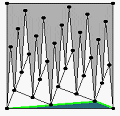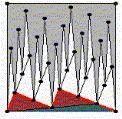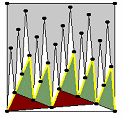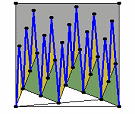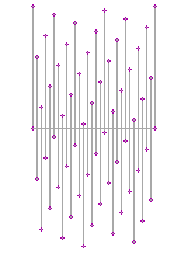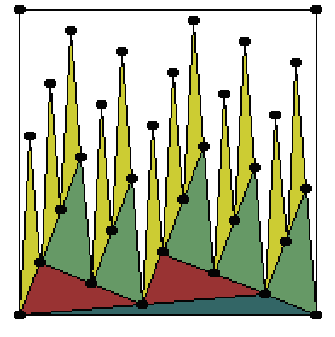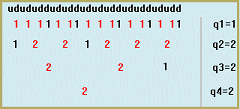Except in the stratified nature of the U/D-series , the stratified nature of the shiftpattern can also be made explicit in the scattering-pattern of the rotational axes, applying a geometrical model of stacked triangles to this pattern. In the example the sd-ratio is 12/17. Applying the principle of continued fraction results in 4 q-values: q1=1; q2=2; q3=2; q4=2. Similar, there are 4 strata in the stacked structure of the trangles: - the blue triangle represents the first stratum (q4); - the brown triangles, added to the blue, represent the second stratum (q3); - the green triangles, added to the former two types, represent the third stratum (q2); - the yellow triangles, added to the other three types, represent the fourth stratum (q1). Each stratum is represented by a path which is composed of more slanting and less slanting lines which alternate. The most slanting lines in all cases consist of but one segment. The less slanting lines are composed of a variable number of segments. This number always ranges between q and q +1. The values q are supplied by the least slanting side of the triangles which represent the concerning stratum; the values 1 are supplied by the most slanting side of the triangles which represents the stratum one position lower. The successive lengths of the least slanting lines in a path represent the complexity of the referring stratum. This complexity can be represented by a series in which the symbols q and 1 alternate. The various series equal the series in the numerical mode.l